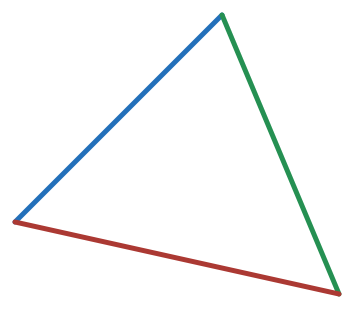# Apply Generating Functions

Geometry Level 3Find the number of distinct triplets $(a,b,c)$ integers such that $a and $a,b,c$ are the sides of triangle whose perimeter is $21$.

Check out my set Some JEE problems

×